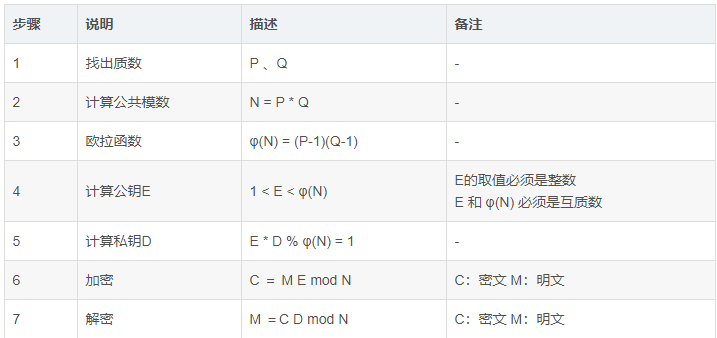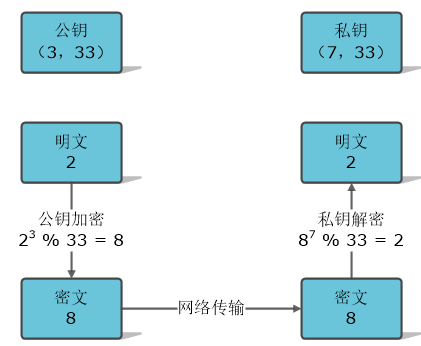# RSA 非对称加密原理，小白速来

RSA 加密原理## 示例

1、找出质数 P 、Q

P = 3
Q = 11

2、计算公共模数

N = P * Q = 3 * 11 = 33
N = 33

3、 欧拉函数

φ(N) = (P-1)(Q-1) = 2 * 10 = 20
φ(N) = 20

4、计算公钥E

1 < E < φ(N)
1 <E < 20

E 的取值范围 {3, 7, 9, 11, 13, 17, 19}
E的取值必须是整数, E 和 φ(N) 必须是互质数

3 和 φ(N) =20 互为质数，满足条件

5、计算私钥D

E * D % φ(N) = 1
3 * D  % 20 = 1   

6、公钥加密

M = 2
E = 3
N = 33

C = 2^3 % 33 = 8

7、私钥解密

M ＝C^D mod N

C = 8
D = 7
N = 33

M = 8^7 % 33
8 * 8 * 8 * 8 * 8 * 8 * 8=2097152
8 * 8 * 8 * 8 * 8 * 8 * 8 % 33 = 2

## 公钥加密 - 私钥解密流程图## 私钥加密 - 公钥解密流程图09-131114
12-1947206-055万+
01-26665
11-28206
09-194009
07-22120
08-17964
09-054411
10-23157